# HEAT TRANSFER

Heat is the form of energy which flows from one body to another body in the form of radiation, molecular vibrations and molecular displacement. The mode of heat flow from this method are collectively known as heat transfer.
*. There are three mode of heat transfer.
1). Conduction
2). Convection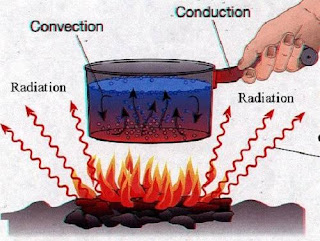*. THERMAL CONDUCTIVITY – It is the ability of the material to conduct heat from them.
*. HEAT CONDUCTION – It is time rate of flow of heat through any material for a given temperature difference.
*. The rate of heat transfer through a metal rod of length L and cross sectional area A and the temperature at the ends are T1 and T2 is –
Rate of heat transfer = ∆Q/∆t = KA(T1-T2)/L
H = KA∆T/L and also H = Q/t
So then Q = KA∆T.t/L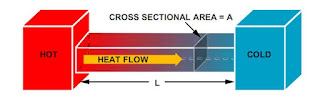Where K is called the cofficient of thermal conductivity.
SI unit of K is J/smK or W/mK
The term ∆T/L is called temperature gradient.
*. The rate of flow of heat = heat current or thermal current, and it is denoted by H.
H = ∆Q/∆t = KA(T1-T2)/L = T1-T2/(L/KA)
therma resistance = L/KA = ∆T/H = T1-T2/H
R = L/KA

### CONNECTION OF ROD WITH DIFFERENT THERMAL CONDUCTIVITIES

SERIES CONNECTION OF TWO THERMAL CONDUCTORS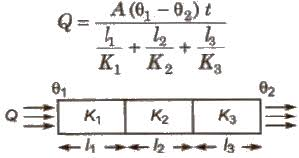*. Equivalent resistance = R(eq) = R1 + R2
*. Equivalent thermal conductivity if their length are equal= K(eq) = 2K1K2/K1+K2
PARALLEL CONNECTION OF TWO THERMAL CONDUCTORS
*. Equivalent resistance = 1/R(eq)= 1/R1 + 1/R2
*. Equivalent thermal conductivity if their cross sectional area are equal = K(eq) = K1+K2/2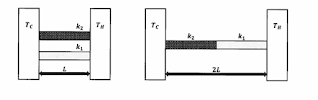### CONVECTION

*. It is the mode of heat transfer in which heat transfer occurs due to molecular displacement from high temperature region to the low temperature region. This mode of heat transfer usually takes place in liquids.
*. Application of convection in daily life
1). In boiling of water.
2). Formation of land and sea breeze.
3). The invariable 4°C temperature of the bottom of the deep sea whether it is summer or winter.
4). Illumination of room with candle , but not the interior of a free falling lift or satellite.
5). Heating of atmosphere.

*. It is also a mode of heat transfer in which heat is transferred from one place to another place without heating the intervening medium.
*. In the context of heat transfer, radiation is the process, in which energy is emitted by a body who travelled through space and falls on a body.
But in electromagnetism, radiation is the electromagnetic waves which carry energy by itself.

*. All objects emit radiation simply, whose temperature is just above the obsolute zero and all objects absorbs some of the radiation which falls on them .
*. According to the electromagnetic theory Maxwell shows that all radiation are the electromagnetic waves and their source are the vibrations of the charged particles.
*. If the tempt of a object is high then more radiation emit if tempt is low then less radiation emit.
*. The radiations which emit on the high temperature their wavelength is short in comparison with the wavelength of radiation which emit on the low temperature.
*. All thermal radiation travels with the speed of light.
*. All radiation are the electromagnetic waves and can travel through space or vaccum.
*. Just similar to light, radiation can also be reflected , refracted, diffracted and polarised.
*. All radiation from their point source obeys inverse square law.

### PREVOST THEORY OF EXCHANGE

*. According to this theory, all objects radiate thermal radiation at all tempt.
*. The amount of thermal radiation radiated per unit time depends on the nature of the object , surface area and it’s temperature.
*. The rate of emitting of thermal radiation is higher in high temperature body.

### PERFECTLY BLACK BODY AND BLACK BODY RADIATION

*. PERFECTLY BLACK BODY – It is body which absorbs all the heat radiations of every wavelength , which are incidents on it. This body doesn’t reflect and transmit the radiation so it appears black. Whatever be the colour of the radiation. It just appears black.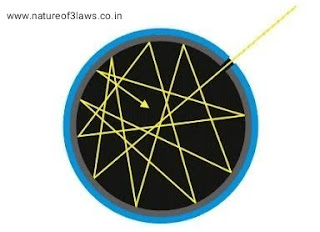*. There is no any perfectly black body found naturally. But lamp black,  platinum black and ferry black are some black bodies which are nearly perfectly black. They absorbs about 99% of incidents radiation.

#### ABSORPTION , REFLECTION , AND EMISSIONS OF RADIATION

*. If Q = Qr + Qt + Qa and after dividing by Q both side we get, 1 = Qr/Q + Qt/Q + Qa/Q
Where r = reflective power
t = transmission power
a = absorptive power
If r= 0 , t=0 , a = 1 then it will be perfectly black body.
If r= 0 , t= 1 , a= 0 then it will be perfect transmitter
If r=1 , t=0 ,a=0 then it will be perfect reflector

#### ABSORPTIVE POWER

*. It is the ratio of the energy absorb to the energy incident.
a = energy absorb / energy incident

#### EMISSIVE POWER

*. It is the energy radiated per unit time and per unit area along the normal of the area.
E = Q/∆A.∆t
*. Emissivity – It is the ratio of the emissive power of a body at temperature T to the emissive power of a black body at same temperature. It is denoted by ε.

#### SPECTRAL EMISSIVE POWER (Eλ)

*. Spectral emissive power(Eλ) is the emissive power per unit wavelength.
Eλ = dE/dλ

#### KIRCHHOFF’S LAW

*. This laws states that the ratio of the emissive power to the absorptive power of a radiation whose wavelength is given is the same for all objects at the same temperature and it is equal to the emissive power of a perfectly black body for the same temperature and the wavelength.
E(body)/a(body) = E(perfectly black body)
*. Good absorber of a particular wavelength λ is also a good emitter of the same wavelength.

#### STEFAN – BOLTZMANN’ S LAW

*. This law states that in a black body the amount of radiation radiated per unit time from a area A at absolute temperature T is directly proportional to the fourth power of the temperature.
u = σAT^4
where σ is the proportionality constant called Stefan constant and its value is =
5.67×10^-8W/m^2.K^4
*. If emissivity of a body is given then the amount of radiation radiated per unit time is
u = εσAT^4
*. If the surrounding temperature is T0 then the net amount of energy radiated by area A in per unit time is ,
∆u = u – u0 = εσA(T^4 – T0^4)

#### NEWTON’S  LAW OF COOLING

*. This law states that the rate of cooling of a body is directly proportional to the temperature difference and area exposed.
dQ/dt = -k(Ti – T0) ,where Ti and T0 is the temperature of the body and the surroundings.
*. Temperature of the body at any instant of time t is given as-
T = T0 + (Ti -T0)e^-kt , where k is constant.
*. The value of k can be directly find from the Stefan law and it can be given as
k = 4εσT0^3A/ms
*. If Qemission < Qabsorption , then the body become hotter.
If Qemission>Qabsorption, then the body become colder.
IfQabsorption=Qabsorption , then the body remains constant( thermal equilibrium attained)

#### WIEN’S DISPLACEMENT LAW

*. This law states that the wavelength λm corresponding to the maximum intensity of the emission decreases inversely with the increase in the temperature of the black body.Here b is called the wien’s constant and its value is b = 0.282cm-K*. Higher temperatures of the body  , means higher area under the curves.
*. High amount of energy is radiated if the temperature of the body is higher.
*. Energy emitted by the body at temperatures is not uniform , for long and short wavelength energy emission is low.
*. For a given temperature there is a particular wavelength λm, for which energy emission Eλ is maximum.
*. Maxima of the curve shift towards the shorter wavelength if temperature is increased in black body.